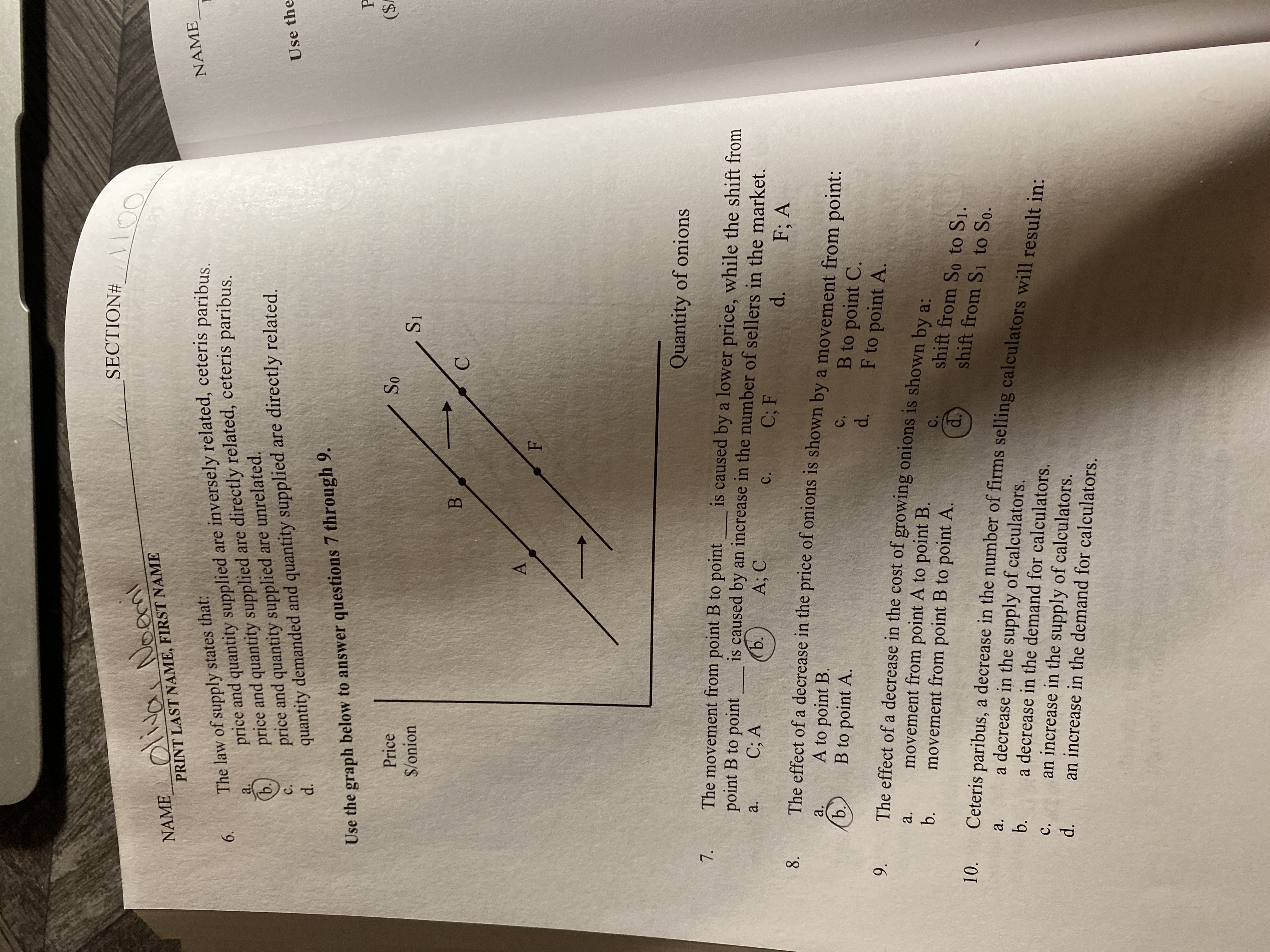# SECTION# _ \ on100NAME__O\\ NoemPRINT LAST NAME, FIRST NAMEThe law of supply states that:price and quantity supplied are inversely related, ceteris paribus.price and quantity supplied are directly related, ceteris paribus.NAMEa.ь.price and quantity supplied are unrelated.quantity demanded and quantity supplied are directly related.C.Use thed.Use the graph below to answer questions 7 through 9.Price(\$So\$/onionS1Quantity of onionsThe movement from point B to pointpoint B to pointC; Ais caused by a lower price, while the shift fromis caused by an increase in the number of sellers in the market.ъ.a.A; CC; FC.d.F; AThe effect of a decrease in the price of onions is shown by a movement from point:8.A to point B.B to point A.a.b.C.B to point C.F to point A.d.The effect of a decrease in the cost of growing onions is shown by a:movement from point A to point B.movement from point B to point A.9.a.b.C.shift from So to S1.d.shift from S1 to So.Ceteris paribus, a decrease in the number of firms selling calculators will result in:10.a decrease in the supply of calculators.a decrease in the demand for calculators.an increase in the supply of calculators.an increase in the demand for calculators.a.b.C.d.6.7.

Question
11 views

Question 9help_outlineImage TranscriptioncloseSECTION# _ \ on 100 NAME__O\\ Noem PRINT LAST NAME, FIRST NAME The law of supply states that: price and quantity supplied are inversely related, ceteris paribus. price and quantity supplied are directly related, ceteris paribus. NAME a. ь. price and quantity supplied are unrelated. quantity demanded and quantity supplied are directly related. C. Use the d. Use the graph below to answer questions 7 through 9. Price (\$ So \$/onion S1 Quantity of onions The movement from point B to point point B to point C; A is caused by a lower price, while the shift from is caused by an increase in the number of sellers in the market. ъ. a. A; C C; F C. d. F; A The effect of a decrease in the price of onions is shown by a movement from point: 8. A to point B. B to point A. a. b. C. B to point C. F to point A. d. The effect of a decrease in the cost of growing onions is shown by a: movement from point A to point B. movement from point B to point A. 9. a. b. C. shift from So to S1. d. shift from S1 to So. Ceteris paribus, a decrease in the number of firms selling calculators will result in: 10. a decrease in the supply of calculators. a decrease in the demand for calculators. an increase in the supply of calculators. an increase in the demand for calculators. a. b. C. d. 6. 7. fullscreen
check_circle

Step 1

Supply Curve: It is the graphical object showing the relationship between the price of a good and the...

### Want to see the full answer?

See Solution

#### Want to see this answer and more?

Solutions are written by subject experts who are available 24/7. Questions are typically answered within 1 hour.*

See Solution
*Response times may vary by subject and question.
Tagged in